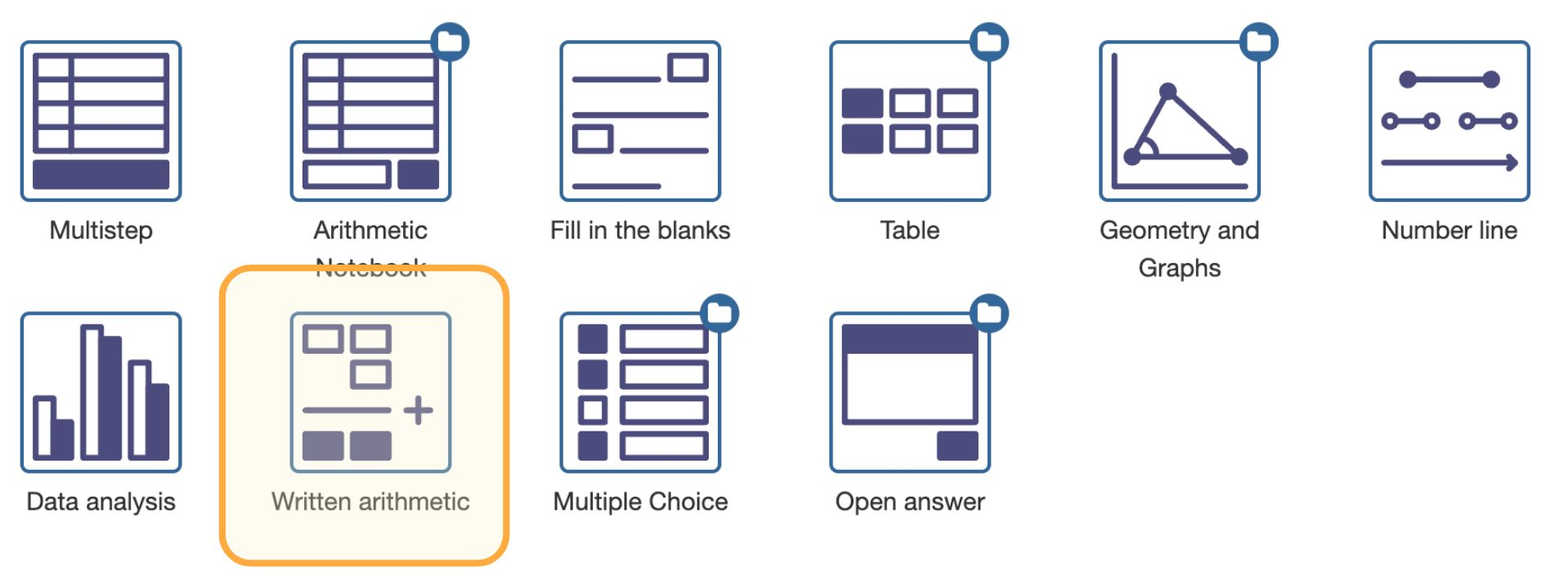# Written ArithmeticFigure: Selecting the Written Arithmetic question type.

Written Arithmetic is a question type for multi-digit addition, subtraction, multiplication, and division. See here for a general description of this question type.

In this section we explain how you create new exercises using Written Arithmetic. You will see that you have to do little more than specifying the numbers to add (or multiply, subtract, divide) and set some configuration options. Everything else is handled automatically.